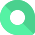# ARR Growth Rate

Date created: Apr 7, 2021  •   Last updated: Jul 5, 2021

## What is ARR Growth Rate?

ARR Growth Rate is the change in annual recurring revenue over a given period, typically represented in a percentage.

Alternate names: Annual Recurring Revenue Growth Rate

### Formula

ƒ (Sum(ARR end of period) – Sum(ARR beginning of period))/Sum(ARR beginning of period)
ƒ (Sum(ARR end of period)/Sum(ARR beginning of period)) - 1

### How to calculate

A SaaS business saw an increase in ARR from the previous year at \$2 million to \$3.5 million ARR at the end of this year. The ARR Growth Rate for this business at the end of this year is (\$3.5m - \$2m) / \$2m which is 75%.

ARR Growth Rate

### Start tracking your ARR Growth Rate data

Use Klipfolio PowerMetrics, our free analytics tools, to monitor your data.

### How does this work?Step 2Create your free Klipfolio PowerMetrics Account### What is a good ARR Growth Rate benchmark?

The median ARR Growth Rate is 41% for companies with an ARR between \$2.5 million and \$10 million.

YoY Growth Rate by ARR

OpenView, 2020